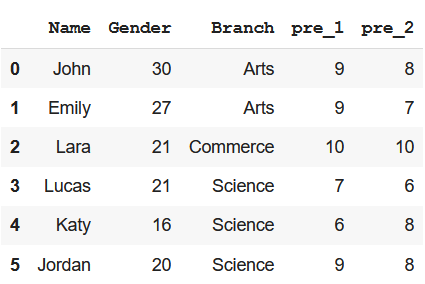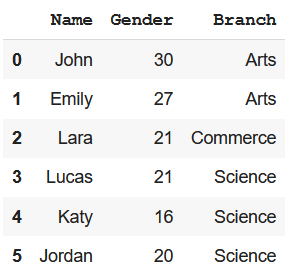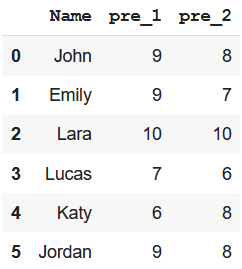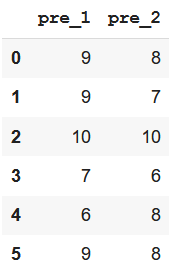Skip to content
Related Articles
Python – Subset DataFrame by Column Name
• Last Updated : 16 Mar, 2021

Using Pandas library, we can perform multiple operations on a DataFrame. We can even create and access the subset of a DataFrame in multiple formats. The task here is to create a subset DataFrame by column name. We can choose different methods to perform this task. Here are possible methods mentioned below –

Before performing any action, we need to write few lines of code to import necessary libraries and create a DataFrame.

Creating the DataFrame

## Python3

 `#import pandas``import` `pandas as pd`` ` `# create dataframe``data ``=` `{``'Name'``: [``'John'``, ``'Emily'``, ``'Lara'``, ``'Lucas'``, ``'Katy'``, ``'Jordan'``], ``        ``'Gender'``: [``30``, ``27``, ``21``, ``21``, ``16``, ``20``], ``        ``'Branch'``: [``'Arts'``, ``'Arts'``, ``'Commerce'``, ``'Science'``, ``                   ``'Science'``, ``'Science'``],``        ``'pre_1'``: [``9``, ``9``, ``10``, ``7``, ``6``, ``9``],``        ``'pre_2'``: [``8``, ``7``, ``10``, ``6``, ``8``, ``8``]}`` ` `df ``=` `pd.DataFrame(data)``df`

Output:Method 1: Using Python iloc() function

This function allows us to create a subset by choosing specific values from columns based on indexes.

Syntax:

`df_name.iloc[beg_index:end_index+1,beg_index:end_index+1]`

Example: Create a subset with Name, Gender and Branch column

## Python3

 `# create a subset of all rows``# and Name, Gender and Branch column``df.iloc[:, ``0``:``3``]`

Output :Method 2: Using Indexing Operator

We can use the indexing operator i.e. square brackets to create a subset dataframe

Example: Create a subset with Name, pre_1, and pre_2 column

## Python3

 `# creating subset dataframe using ``# indexing operator``df[[``'Name'``, ``'pre_1'``, ``'pre_2'``]]`

Output –Method 3: Using filter() method with like keyword

We can use this method particularly when we have to create a subset dataframe with columns having similarly patterned names.

Example: Create a subset with pre_1 and pre_2 column

## Python3

 `# create a subset of columns pre_1 and pre_2``# using filter() method``df.``filter``(like``=``'pre'``)`

Output:Attention geek! Strengthen your foundations with the Python Programming Foundation Course and learn the basics.

To begin with, your interview preparations Enhance your Data Structures concepts with the Python DS Course. And to begin with your Machine Learning Journey, join the Machine Learning – Basic Level Course

My Personal Notes arrow_drop_up